# b632 method To Binomial distribution (Statistics)

### b632 method:

A procedure of error rate estimation in discriminant analysis based on the bootstrap, which consists of the following steps:

(1) Randomly sample (with replacement) a bootstrap sample from the original data.

(2) Classify the observations omitted from the bootstrap sample using the classification rule calculated from the bootstrap sample.

(3) Repeat (1) and (2) many times and calculate the mean bootstrap classification matrix, Cb.

(4) Calculate the resubstitution classification matrix, Cr, based on the original data.

(5) The b632 estimator of the classification matrix is 0.368Cr + 0.632Q, from which the required error rate estimate can be obtained.

### Bk method:

A form of cluster analysis which produces overlapping clusters. A maximum of k — 1 objects may belong to the overlap between any pair of clusters. When k = 1 the procedure becomes single linkage clustering.

### Babbage, Charles (1792-1871):

Born near Teignmouth in Devon, Babbage read mathematics at Trinity College, Cambridge, graduating in 1814. His early work was in the theory of functions and modern algebra. Babbage was elected a Fellow of the Royal Society in 1816. Between 1828 and 1839 he held the Lucasian Chair of Mathematics at Trinity College. In the 1820s Babbage developed a ‘Difference Engine’ to form and print mathematical tables for navigation and spent much time and money developing and perfecting his calculating machines. His ideas were too ambitious to be realized by the mechanical devices available at the time, but can now be seen to contain the essential germ of today’s electronic computer. Babbage is rightly seen as the pioneer of modern computers.

### Back-calculation:

Synonym for back-projection.

### Back-projection:

A term most often applied to a procedure for reconstructing plausible HIV incidence curves from AIDS incidence data. The method assumes that the probability distribution of the incubation period of AIDS has been estimated precisely from separate cohort studies and uses this distribution to project the AIDS incidence data backwards to reconstruct an HIV epidemic curve that could plausibly have led to the observed AIDS incidence data.

### Back-to-back stem-and-leaf plots:

A method for comparing two distributions by ‘hanging’ the two sets of leaves in the stem-and-leaf plots of the two sets of data, off either side of the same stem. An example appears in Fig. 9.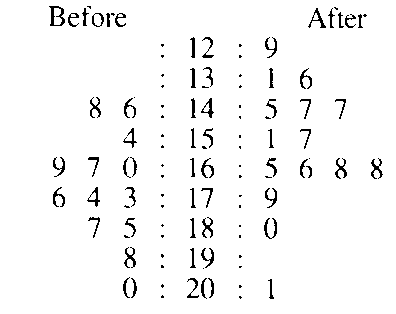Fig. 9 Back-to-back stem-and-leaf plot of systolic blood pressure of fifteen subjects before and two hours after taking the drug captoril.

### Backward-looking study:

An alternative term for retrospective study.

### Backward shift operator:

A mathematical operator denoted by B, met in the analysis of time series. When applied to such a series the operator moves the observations back one time unit, so that if xt represents the values of the series then, for example,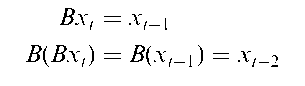### Bagging:

A term used for producing replicates of the training set in a classification problem and producing an allocation rule on each replicate. The basis of bagging predictors which involve multiple versions of a predictor that are used to get an aggregated predictor.

### Bagplot:

An approach to detecting outliers in bivariate data. The plot visualizes location, spread, correlation, skewness and the tails of the data without making assumptions about the data being symmetrically distributed.

### Balaam’s design:

A design for testing differences between two treatments A and B in which patients are randomly allocated to one of four sequences, AA, AB, BA, or BB. See also crossover design.

### Balanced design:

A term usually applied to any experimental design in which the same number of observations is taken for each combination of the experimental factors.

### Balanced incomplete block design:

A design in which not all treatments are used in all blocks. Such designs have the following properties:

• each block contains the same number of units;

• each treatment occurs the same number of times in all blocks;

• each pair of treatment combinations occurs together in a block the same number of times as any other pair of treatments.

In medicine this type of design might be employed to avoid asking subjects to attend for treatment an unrealistic number of times, and thus possibly preventing problems with missing values. For example, in a study with five treatments, it might be thought that subjects could realistically only be asked to make three visits. A possible balanced incomplete design in this case would be the following:

 Patient Visit 1 Visit 2 Visit 3 1 T4 T5 Ti 2 T4 T2 T5 3 T2 T4 Ti 4 T5 T3 Ti 5 T3 T4 T5 6 T2 T3 Ti 7 T3 Ti T4 8 T3 T5 T2 9 T2 T3 T4 10 T5 Ti T2

### Balanced incomplete repeated measures design (BIRMD):

An arrangement of N randomly selected experimental units and k treatments in which every unit receives k1 treatments 1 < k1 < k, each treatment is administered to r experimental units and each pair of treatments occurs together X times. See also balanced incomplete blocks.

### Balanced repeated replication (BRR):

A popular method for variance estimation in surveys which works by creating a set of ‘balanced’ pseudoreplicated datasets from the original dataset. For an estimator, 0, of a parameter, 0, the estimated variance is obtained as the average of the squared deviations, 6(r) — 6, where 0(r) is the estimate based on the rth replicated data set. See also jackknife.

### Balancing score:

Synonymous with propensity score.

### Ballot theorem:

Let X1, X2,…, Xn be independent random variables each with a Bernoulli distribution with Pr(X, = 1) = Pr(X, = — 1) = 2. Define Sk as the sum of the first k of the observed values of these variables, i.e. Sk = X1 + X2 + ••• + Xk and let a and b be nonnegative integers such that a — b > 0 and a + b = n, then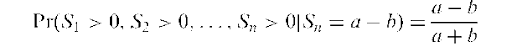If +1 is interpreted as a vote for candidate A and —1 as a vote for candidate B, then Sk is the difference in numbers of votes cast for A and B at the time when k votes have been recorded; the probability given is that A is always ahead of B given that A receives a votes in all and B receives b votes. [An Introduction to Probability Theory and its Applications, Volume 1, 3rd edition, 1968, W. Feller, Wiley, New York.]

### BAN:

Abbreviation for best asymptotically normal estimator.

### Banach’s match-box problem:

A person carries two boxes of matches, one in their left and one in their right pocket. Initially they contain N matches each. When the person wants a match, a pocket is selected at random, the successive choices thus constituting Bernoulli trials with p = 2. On the first occasion that the person finds that a box is empty the other box may contain 0, 1, 2,…, N matches. The probability distribution of the number of matches, R, left in the other box is given by: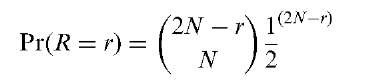So, for example, for N = 50 the probability of there being not more than 10 matches in the second box is 0.754. [An Introduction to Probability Theory and its Applications, Volume 1, 3rd edition, 1968, W. Feller, Wiley, New York.]

### Bancroft, Theodore Alfonso (1907-1986):

Born in Columbus, Mississippi, Bancroft received a first degree in mathematics from the University of Florida. In 1943 he completed his doctorate in mathematical statistics with a dissertation entitled ‘Tests of Significance Considered as an Aid in Statistical Methodology’. In 1950 he became Head of the Department of Statistics of the Iowa Agriculture and Home Economics Experiment Station. His principal area of research was incompletely specified models. Bancroft served as President of the American Statistical Association in 1970. He died on 26 July 1986 in Ames, Iowa.

### Bar chart:

A form of graphical representation for displaying data classified into a number of (usually unordered) categories. Equal-width rectangular bars are constructed over each category with height equal to the observed frequency of the category as shown in Fig. 10. See also histogram and component bar chart.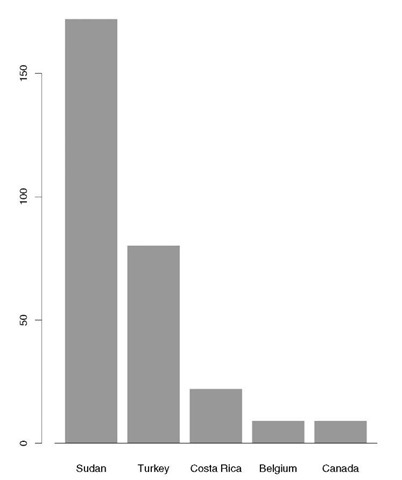Fig. 10 Bar chart of mortality rates per 1000 live births for children under five years of age in five different countries.

### Barnard, George Alfred (1915-2002):

Born in Walthamstow in the east end of London, Barnard gained a scholarship to St. John’s College, Cambridge, where he graduated in mathematics in 1936. For the next three years he studied mathematical logic at Princeton, New Jersey, and then in 1940 joined the engineering firm, Plessey. After three years acting as a mathematical consultant for engineers, Barnard joined the Ministry of Supply and it was here that his interest in statistics developed. In 1945 he went to Imperial College London, and then in 1966 he moved to a chair in the newly created University of Essex, where he stayed until his retirement in 1975. Barnard made major and important contributions to several fundamental areas of inference, including likelihood and 2 x 2 tables. He was made President of the Royal Statistical Society in 1971-2 and also received the Society’s Guy medal in gold. He died in Brightlingsea, Essex, on 30 July 2002.

## Barrett and Marshall model for conception:

A biologically plausible model for the probability of conception in a particular menstrual cycle, which assumes that batches of sperm introduced on different days behave independently. The model is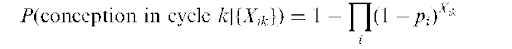where the Xik are 0,1 variables corresponding to whether there was intercourse or not on a particular day relative to the estimated day of ovulation (day 0). The parameter pi is interpreted as the probability that conception would occur following intercourse on day i only. See also EU model.

### Bartholomew’s likelihood function:

The joint probability of obtaining the observed known-complete survival times as well as the so-far survived measurements of individuals who are still alive at the date of completion of the study or other endpoint of the period of observation.

### Bartlett, Maurice Stevenson (1910-2002):

Born in Chiswick, London, Bartlett won a scholarship to Latymer Upper School, where his interest in probability was awakened by a chapter on the topic in Hall and Knight’s Algebra. In 1929 he went to Queen’s College, Cambridge to read mathematics, and in his final undergraduate year in 1932 published his first paper (jointly with John Wishart), on second-order moments in a normal system. On leaving Cambridge in 1933 Bartlett became Assistant Lecturer in the new Statistics Department at University College London, where his colleagues included Egon Pearson, Fisher and Neyman. In 1934 he joined Imperial Chemical Industries (ICI) as a statistician. During four very creative years Bartlett published some two-dozen papers on topics as varied as the theory of inbreeding and the effect of non-normality on the ^-distribution. From ICI he moved to a lectureship at the University of Cambridge, and then during World War II he was placed in the Ministry of Supply. After the war he returned to Cambridge and began his studies of time series and diffusion processes. In 1947 Bartlett was given the Chair of Mathematical Statistics at the University of Manchester where he spent the next 13 years, publishing two important topics, An Introduction to Stochastic Processes (in 1955) and Stochastic Population Models in Ecology and Epidemiology (in 1960) as well as a stream of papers on stochastic processes, etc. It was in 1960 that Bartlett returned to University College taking the Chair in Statistics, his work now taking in stochastic path integrals, spatial patterns and multivariate analysis. His final post was at Oxford where he held the Chair of Biomathematics from 1967 until his retirement eight years later. Bartlett received many honours and awards in his long and productive career, including being made a Fellow of the Royal Society in 1961 and being President of the Royal Statistical Society for 1966-7. He died on 8 January 2002, in Exmouth, Devon.

A correction term for the likelihood ratio that makes the chi-squared distribution a more accurate approximation to its probability distribution.

### Bartlett’s identity:

A matrix identity useful in several areas of multivariate analysis and given by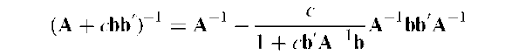where A is q x q and nonsingular, b is a q x 1 vector and c is a scalar.

### Bartlett’s test for eigenvalues:

A large-sample test for the null hypothesis that the last (q — k) eigenvalues, Xk+1,…, Xq, of a variance-covariance matrix are zero. The test statistic is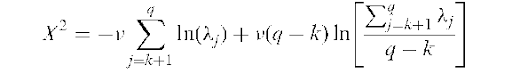Under the null hypothesis, X2 has a chi-squared distribution with (1 =2)(q — k — 1)(q — k + 2) degrees of freedom, where v is the degrees of freedom associated with the covariance matrix. Used mainly in principal components analysis. [MV1 Chapter 4.]

### Bartlett’s test for variances:

A test for the equality of the variances of a number (k) of populations. The test statistic is given by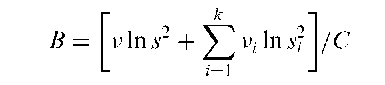where s2 is an estimate of the variance of population i based on v, degrees of freedom, and v and s2 are given by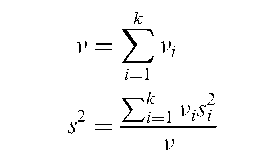and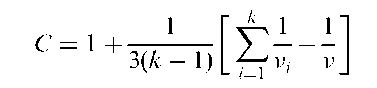Under the hypothesis that the populations all have the same variance, B has a chi-squared distribution with k — 1 degrees of freedom. Sometimes used prior to applying analysis of variance techniques to assess the assumption of homogeneity of variance. Of limited practical value because of its known sensitivity to non-normality, so that a significant result might be due to departures from normality rather than to different variances. See also Box’s test and Hartley’s test.

### Baseline balance:

A term used to describe, in some sense, the equality of the observed baseline characteristics among the groups in, say, a clinical trial. Conventional practice dictates that before proceeding to assess the treatment effects from the clinical outcomes, the groups must be shown to be comparable in terms of these baseline measurements and observations, usually by carrying out appropriate significant tests. Such tests are frequently criticized by statisticians who usually prefer important prognostic variables to be identified prior to the trial and then used in an analysis of covariance.

### Baseline characteristics:

Observations and measurements collected on subjects or patients at the time of entry into a study before undergoing any treatment. The term can be applied to demographic characteristics of the subject such as sex, measurements taken prior to treatment of the same variable which is to be used as a measure of outcome, and measurements taken prior to treatment on variables thought likely to be correlated with the response variable. At first sight, these three types of baseline seem to be quite different, but from the point-of-view of many powerful approaches to analysing data, for example, analysis of covariance, there is no essential distinction between them.

### BASIC:

Acronym for Beginners All-Purpose Symbolic Instruction Code, a programming language once widely used for writing microcomputer programs.

### Basic reproduction number:

A term used in the theory of infectious diseases for the number of secondary cases which one case would produce in a completely susceptible population. The number depends on the duration of the infectious period, the probability of infecting a susceptible individual during one contact, and the number of new susceptible individuals contacted per unit time, with the consequence that it may vary considerably for different infectious diseases and also for the same disease in different populations.

### Basu’s theorem:

This theorem states that if T is a complete sufficient statistic for a family of probability measures and V is an ancillary statistic, then T and V are independent. The theorem shows the connection between sufficiency, ancillarity and independence, and has led to a deeper understanding of the interrelationship between the three concepts.

### Bathtub curve:

The shape taken by the hazard function for the event of death in human beings; it is relatively high during the first year of life, decreases fairly soon to a minimum and begins to climb again sometime around 45-50. See Fig. 11.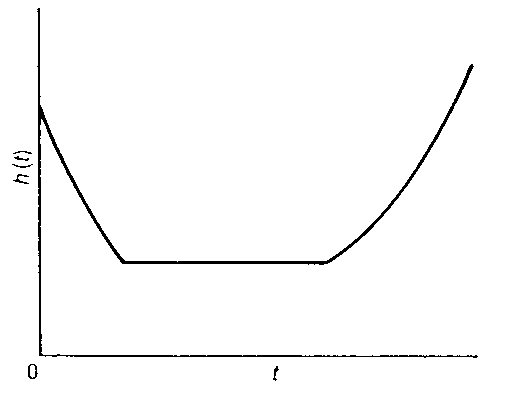Fig. 11 Bathtub curve shown by hazard function for death in human beings.

### Battery reduction:

A general term for reducing the number of variables of interest in a study for the purposes of analysis and perhaps later data collection. For example, an overly long questionnaire may not yield accurate answers to all questions, and its size may need to be reduced. Techniques such as factor analysis and principal component analysis are generally used to achieve the required reduction.

### Bayes factor:

A summary of the evidence for a model M1 against another model M0 provided by a set of data D, which can be used in model selection. Given by the ratio of posterior to prior odds,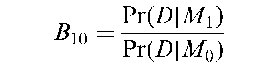Twice the logarithm of B10 is on the same scale as the deviance and the likelihood ratio test statistic. The following scale is often useful for interpreting values of B10;

 2lnB10 Evidence for Mx < 0 Negative (supports M0) 0-2.2 Not worth more than a bare mention 2.2-6 Positive 6-10 Strong > 10 Very strong

Very sensitive to the assumed prior distribution of the parameters.

### Bayes information criterion (BIC):

An index used as an aid to choose between competing statistical models that is similar to Akaike’s information criterion (AIC) but penalizes models of higher dimensionality more than the AIC. Essentially the BIC is equivalent to Schwarz’s criterion. [Journal of the American Statistical Association, 1996, 64, 103-37.]

### Bayesian confidence interval:

An interval of a posterior distribution which is such that the density at any point inside the interval is greater than the density at any point outside and that the area under the curve for that interval is equal to a prespecified probability level. For any probability level there is generally only one such interval, which is also often known as the highest posterior density region. Unlike the usual confidence interval associated with frequentist inference, here the intervals specify the range within which parameters lie with a certain probability.

### Bayesian inference:

An approach to inference based largely on Bayes’ Theorem and consisting of the following principal steps:

(1) Obtain the likelihood, f (x|h) describing the process giving rise to the data x in terms of the unknown parameters h.

(2) Obtain the prior distribution, f (h) expressing what is known about h, prior to observing the data.

(3) Apply Bayes’ theorem to derive the posterior distribution f (h|x) expressing what is known about h after observing the data.

(4) Derive appropriate inference statements from the posterior distribution. These may include specific inferences such as point estimates, interval estimates or probabilities of hypotheses. If interest centres on particular components of h their posterior distribution is formed by integrating out the other parameters.

This form of inference differs from the classical form of frequentist inference in several respects, particularly the use of the prior distribution which is absent from classical inference. It represents the investigator’s knowledge about the parameters before seeing the data. Classical statistics uses only the likelihood. Consequently to a Bayesian every problem is unique and is characterized by the investigator’s beliefs about the parameters expressed in the prior distribution for the specific investigation.

### Bayesian model averaging (BMA):

An approach to selecting important subsets of variables in regression analysis, that provides a posterior probability that each variable belongs in a model; this is often a more directly interpretable measure of variable importance than a p-value.

### Bayesian persuasion probabilities:

A term for particular posterior probabilities used to judge whether a new therapy is superior to the standard, derived from the priors of two hypothetical experts, one who believes that the new therapy is highly effective and another who believes that it is no more effective than other treatments. The persuade the pessimist probability is the posterior probability that the new therapy is an improvement on the standard assuming the sceptical experts prior, and the persuade the optimist probability; is the posterior probability that the new therapy gives no advantage over the standard assuming the enthusiasts prior. Large values of these probabilities should persuade the a priori most opinionated parties to change their views. [Statistics in Medicine, 1997, 16, 1792-802.]

### Bayes’ network:

Essentially an expert system in which uncertainty is dealt with using conditional probabilities and Bayes’ Theorem. Formally such a network consists of the following:

• A set of variables and a set of directed edges between variables.

• Each variable has a finite set of mutually exclusive states.

• The variables together with the directed edges form a conditional independence graph.

• To each variable A with parents Bx,…, Bn there is attached a conditional probability table Pr(AjBx, B2,…, Bn).

An example is shown in Fig. 12.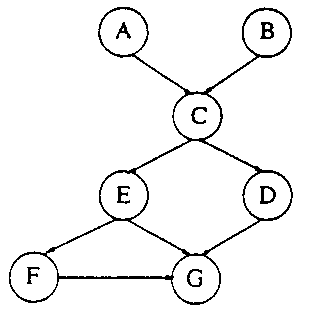Fig. 12 An example of a Bayes’ network.

### Bayes, Reverend Thomas (1702-1761):

Born in London, Bayes was one of the first six Nonconformist ministers to be publicly ordained in England. Reputed to be a skilful mathematician although oddly there is no sign of him having published any scientific work before his election to the Royal Society in 1741. Principally remembered for his posthumously published Essay Towards Solving a Problem in the Doctrine of Chance which appeared in 1763 and, heavily disguised, contained a version of what is today known as Bayes’ Theorem. Bayes died on 7 April 1761 in Tunbridge Wells, England.

### Bayes’ Theorem:

A procedure for revising and updating the probability of some event in the light of new evidence. The theorem originates in an essay by the Reverend Thomas Bayes. In its simplest form the theorem may be written in terms of conditional probabilities as,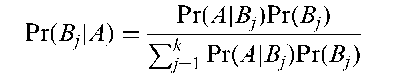where Pr(A|Bj) denotes the conditional probability of event A conditional on event Bj and B1, B2,…, Bk are mutually exclusive and exhaustive events. The theorem gives the probabilities of the Bj when A is known to have occurred. The quantity Pr(Bj) is termed the prior probability and Pr(Bj |A) the posterior probability. Pr(A|Bj) is equivalent to the (normalized) likelihood, so that the theorem may be restated as posterior a (prior)x(likelihood) See also Bayesian inference.

### BBR:

Abbreviation for balanced repeated replication.

### BCa:

Abbreviation for bias-corrected percentile interval.

### Beattie’s procedure:

A continous process-monitoring procedure that does not require 100% inspection. Based on a cusum procedure, a constant sampling rate is used to chart the number of percent of nonconforming product against a target reference value.

### Behrens-Fisher problem:

The problem of testing for the equality of the means of two normal distributions that do not have the same variance. Various test statistics have been proposed, although none are completely satisfactory. The one that is most commonly used however is given by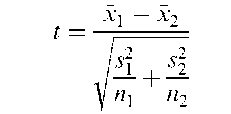where x1, x2, sj, s2, n1 and n2 are the means, variances and sizes of samples of observations from each population. Under the hypothesis that the population means are equal, t has a Student’s t-distribution with v degrees of freedom where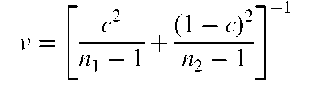and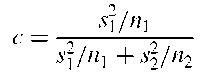### Believe the positive rule:

A rule for combining two diagnostic tests, A and B, in which ‘disease present’ is the diagnosis given if either A or B or both are positive. An alternative believe the negative rule assigns a patient to the disease class only if both A and B are positive. These rules do not necessarily have better predictive values than a single test; whether they do depends on the association between test outcomes.

### Bellman-Harris process:

An age-dependent branching process in which individuals have independent, identically distributed lifespans, and at death split into independent identically distributed numbers of offspring.

### Bell-shaped distribution:

A probability distribution having the overall shape of a vertical cross-section of a bell. The normal distribution is the most well known example, but Student’s -distribution is also this shape.

### Benchmarking:

A procedure for adjusting a less reliable series of observations to make it consistent with more reliable measurements or benchmarks. For example, data on hospital bed occupation collected monthly will not necessarily agree with figures collected annually and the monthly figures (which are likely to be less reliable) may be adjusted at some point to agree with the more reliable annual figures. See also Denton method. [International Statistical Review, 1994, 62, 365-77.]

### Bench-mark dose:

A term used in risk assessment studies where human, animal or ecological data are used to set safe low dose levels of a toxic agent, for the dose that is associated with a particular level of risk.

### Benini, Rodolpho (1862-1956):

Born in Cremona, Italy, Rodolpho was appointed to the Chair of History of Economics at Bari at the early age of 27. From 1928 to his death in 1956 he was Professor of Statistics at Rome University. One of the founders of demography as a separate science.

### Benjamin, Bernard (1910-2002):

Benjamin was educated at Colfe’s Grammar School in Lewisham, South London, and later at Sir John Cass College, London, where he studied physics. He began his working life as an actuarial assistant to the London County Council pension fund and in 1941 qualified as a Fellow of the Institute of Actuaries. After World War II he became Chief Statistician at the General Register Office and was later appointed as Director of Statistics at the Ministry of Health. In the 1970s Benjamin joined City University, London as the Foundation Professor of Actuarial Science. He published many papers and topics in his career primarily in the areas of actuarial statistics and demography. Benjamin was made President of the Royal Statistical Society from 1970-2 and received the society’s highest honour, the Guy medal in gold, in 1986.

### Benjamini and Hochberg step-up methods:

Methods used in bioinformatics to control the false discovery rate when calculating p values from g tests under g individual null hypotheses, one for each gene.

### Bentler-Bonnett index:

A goodness of fit measure used in structural equation modelling.

### Berkson, Joseph (1899-1982):

Born in New York City, Berkson studied physics at Columbia University, before receiving a Ph.D. in medicine from Johns Hopkins University in 1927, and a D.Sc. in statistics from the same university in 1928. In 1933 he became Head of Biometry and Medical Statistics at the Mayo Clinic, a post he held until his retirement in 1964. His research interests covered all aspects of medical statistics and from 1928 to 1980 he published 118 scientific papers. Involved in a number of controversies particularly that involving the role of cigarette smoking in lung cancer, Berkson enjoyed a long and colourful career. He died on 12 September 1982 in Rochester, Minnesota.

### Berkson’s fallacy:

The existence of artifactual correlations between diseases or between a disease and a risk factor arising from the interplay of differential admission rates from an underlying population to a select study group, such as a series of hospital admissions. In any study that purports to establish an association and where it appears likely that differential rates of admission apply, then at least some portion of the observed association should be suspect as attributable to this phenomenon. See also Simpson’s paradox and spurious correlation. [SMR Chapter 5.]

### Bernoulli distribution:

The probability distribution of a binary random variable, X, where Pr(X = 1)=p and Pr(X = 0) = 1 – p. Named after Jacques Bernoulli (1654-1705). All moments of X about zero take the value p and the variance of X is p(1 — p). The distribution is negatively skewed when p > 0.5 and is positively skewed when p < 0.5. [STD Chapter 4.]

### Bernoulli, Jacques (1654-1705) (also known as James or Jakob):

Born in Basel, Switzerland, the brother of Jean Bernoulli and the uncle of Daniel Bernoulli the most important members of a family of Swiss mathematicians and physicists. Destined by his father to become a theologian, Bernoulli studied mathematics in secret and became Professor of Mathematics at Basel in 1687. His topic Ars Conjectandi published in 1713, eight years after his death, was an important contribution to probability theory. Responsible for the early theory of permutations and combinations and for the famous Bernoulli numbers.

### Bernoulli-Laplace model:

A probabilistic model for the flow of two liquids between two containers. The model begins by imagining r black balls and r white balls distributed between two boxes. At each stage one ball is chosen at random from each box and the two are interchanged. The state of the system can be specified by the number of white balls in the first box, which can take values from zero to r. The probabilities of the number of white balls in the first box decreasing by one (pi;i— 1), increasing by one (pii+1) or staying the same (pi t) at the stage when the box contains i white balls, can be shown to be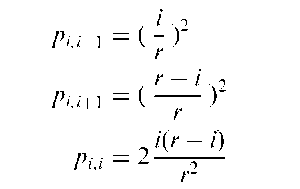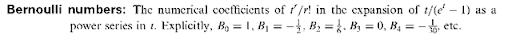### Bernoulli trials:

A set of n independent binary variables in which the jth observation is either a ‘success’ or a ‘failure’, with the probability of success, p, being the same for all trials

### Bernstein polynomial prior:

A nonparametric prior for probability densities on the unit interval.

### Berry-Esseen theorem:

A theorem relating to how rapidly the distribution of the mean approaches normality. See also central limit theorem.

### Bessel function distributions:

A family of probability distributions obtained as the distributions of linear functions of independent random variables, X1 and X2, each having a chi-squared distribution with common degrees of freedom v. For example the distribution of Y = a1 X1 + a2X2 with a1 > 0 and a2 > 0 is f (y) given by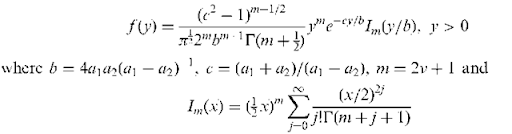### Best asymptotically normal estimator (BAN):

A CAN estimator with minimal asymptotic variance-covariance matrix. The notion of minimal in this context is based on the following order relationship among symmetric matrices: A < B if B — A is nonnegative definite.

### Best linear unbiased estimator (BLUE):

A linear estimator of a parameter that has smaller variance than any similar estimator of the parameter.

### Beta-binomial distribution:

The probability distribution, f(x), found by averaging the parameter, p, of a binomial distribution over a beta distribution, and given by

### Beta-binomial distribution:

The probability distribution, f(x), found by averaging the parameter, p, of a binomial distribution over a beta distribution, and given by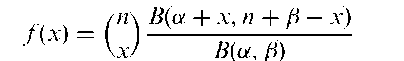where B is the beta function. The mean and variance of the distribution are as follows: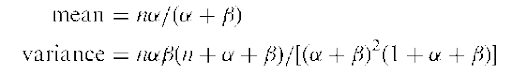Also known as the Polya distribution. For integer a and f, corresponds to the negative hypergeometric distribution. For a = f = 1 corresponds to the discrete rectangular distribution.

### Beta coefficient:

A regression coefficient that is standardized so as to allow for a direct comparison between explanatory variables as to their relative explanatory power for the response variable. Calculated from the raw regression coefficients by multiplying them by the standard deviation of the corresponding explanatory variable.

### Beta distribution:

The probability distribution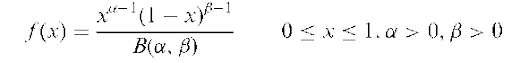where B is the beta function. Examples of the distribution are shown in Figure 13. The mean, variance, skewness and kurtosis of the distribution are as follows: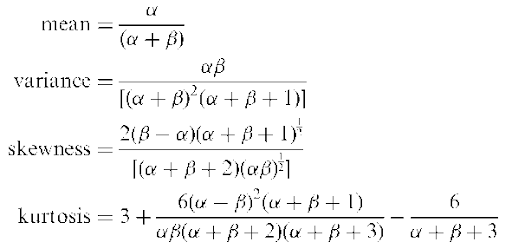A U-shaped distribution if (a – 1)(fi – 1) < 0.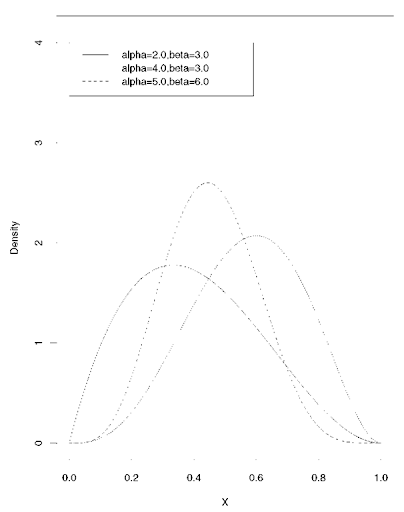Fig. 13 Beta distributions for a number of parameter values.

### Beta(b)-error:

Synonym for type II error.

### Beta function:

The function B(a, p),a> 0, p > 0 given by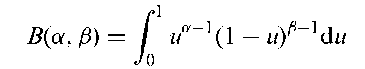Can be expressed in terms of the gamma function T as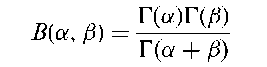The integral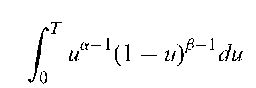is known as the incomplete beta function.

### Beta-geometric distribution:

A probability distribution arising from assuming that the parameter, p, of a geometric distribution has itself a beta distribution. The distribution has been used to model the number of menstrual cycles required to achieve pregnancy.

Between groups matrix of sums of squares and cross-products: See multivariate analysis of variance.

### BGW:

Abbreviation for Bienayme-Galton-Watson process.

### Bhattacharyya bound:

A better (i.e. greater) lower bound for the variance of an estimator than the more well-known Cramer-Rao lower bound.

### Bhattacharya’s distance:

A measure of the distance between two populations with probability distributions f (x) and g(x) respectively. Given by### Bias:

In general terms, deviation of results or inferences from the truth, or processes leading to such deviation. More specifically, the extent to which the statistical method used in a study does not estimate the quantity thought to be estimated, or does not test the hypothesis to be tested. In estimation usually measured by the difference between a parameter estimate 6 and its expected value. An estimator for which E(6) = 6 is said to be unbiased. See also ascertainment bias, recall bias, selection bias and biased estimator.

### Bias-corrected accelerated percentile interval (BCa):

An improved method of calculating confidence intervals when using the bootstrap.

A term that summarizes the fact that if you want less bias in the estimate of a model parameter, it usually costs you more variance. An important concept in the evaluation of the performance of neural networks.

### Biased coin method:

A method of random allocation sometimes used in a clinical trialin an attempt to avoid major inequalities in treatment numbers. At each point in the trial, the treatment with the fewest number of patients thus far is assigned a probability greater than a half of being allocated the next patient. If the two treatments have equal numbers of patients then simple randomization is used for the next patient.

### Biased estimator:

Formally an estimator 6 of a parameter, 6, such that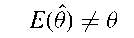The motivation behind using such estimators rather than those that are unbiased, rests in the potential for obtaining values that are closer, on average, to the parameter being estimated than would be obtained from the latter. This is so because it is possible for the variance of such an estimator to be sufficiently smaller than the variance of one that is unbiased to more than compensate for the bias introduced. This possible advantage is illustrated in Fig. 14. The normal curve centred at 6 in the diagram represents the probability distribution of an unbiased estimator of 6 with its expectation equal to 6. The spread of this curve reflects the variance of the estimator. The normal curve centred at E(6) represents the probability distribution of a biased estimator with the bias being the difference between 6 and E(6). The smaller spread of this distribution reflects its smaller variance. See also ridge regression. [ARA Chapter 5.]

### BIC:

Abbreviation for Bayesian information criterion.

### Bienayme-Galton-Watson process (BGW):

A simple branching process defined by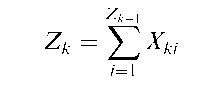where for each k the Xki are independent, identically distributed random variables with the same distribution, pr = Pr(Xj = r), called the offspring distribution.

### Bienayme, Jules (1796-1878):

Born in Paris, Bienayme studied at the Ecole Polytechnique and became lecturer in mathematics at Saint Cyr, the French equivalent of West Point, in 1818. Later he joined the civil service as a general inspector of finance and began his studies of actuarial science, statistics and probability. Made contributions to the theory of runs and discovered a number of important inequalities.

### Big Mac index:

An index that attempts to measure different aspects of the economy by comparing the cost of hamburgers between countries.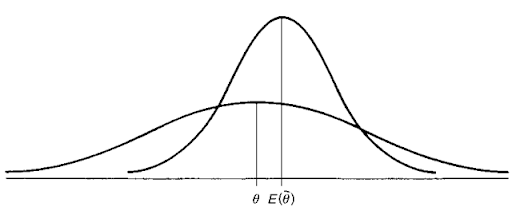Fig. 14 Biased estimator diagram showing advantages of such an estimator.

Bimodal distribution: A probability distribution, or a frequency distribution, with two modes. Figure 15 shows an example of each.

### Binary variable:

Observations which occur in one of two possible states, these often being

labelled 0 and 1. Such data is frequently encountered in medical investigations; commonly occurring examples include ‘dead/alive’, ‘improved/not improved’ and ‘depressed/not depressed.’ Data involving this type of variable often require specialized techniques for their analysis such as logistic regression. See also Bernoulli distribution. [SMR Chapter 2 ]

### Binomial coefficient:

The number of ways that k items can be selected from n items irrespective of their order. Usually denoted C(n, k) or (k) and given by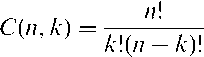### Binomial distribution:

The distribution of the number of ‘successes’, X, in a series of n-independent Bernoulli trials where the probability of success at each trial is p and the probability of failure is q = 1 — p. Specifically the distribution is given by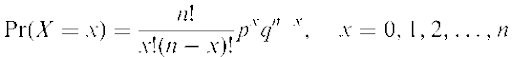The mean, variance, skewness and kurtosis of the distribution are as follows:

mean = np variance = npq

Fig. 15 Bimodal probability and frequency distributions.